# 7.4: Graphing Linear Equations in Two Variables

$$\newcommand{\vecs}{\overset { \rightharpoonup} {\mathbf{#1}} }$$ $$\newcommand{\vecd}{\overset{-\!-\!\rightharpoonup}{\vphantom{a}\smash {#1}}}$$$$\newcommand{\id}{\mathrm{id}}$$ $$\newcommand{\Span}{\mathrm{span}}$$ $$\newcommand{\kernel}{\mathrm{null}\,}$$ $$\newcommand{\range}{\mathrm{range}\,}$$ $$\newcommand{\RealPart}{\mathrm{Re}}$$ $$\newcommand{\ImaginaryPart}{\mathrm{Im}}$$ $$\newcommand{\Argument}{\mathrm{Arg}}$$ $$\newcommand{\norm}{\| #1 \|}$$ $$\newcommand{\inner}{\langle #1, #2 \rangle}$$ $$\newcommand{\Span}{\mathrm{span}}$$ $$\newcommand{\id}{\mathrm{id}}$$ $$\newcommand{\Span}{\mathrm{span}}$$ $$\newcommand{\kernel}{\mathrm{null}\,}$$ $$\newcommand{\range}{\mathrm{range}\,}$$ $$\newcommand{\RealPart}{\mathrm{Re}}$$ $$\newcommand{\ImaginaryPart}{\mathrm{Im}}$$ $$\newcommand{\Argument}{\mathrm{Arg}}$$ $$\newcommand{\norm}{\| #1 \|}$$ $$\newcommand{\inner}{\langle #1, #2 \rangle}$$ $$\newcommand{\Span}{\mathrm{span}}$$$$\newcommand{\AA}{\unicode[.8,0]{x212B}}$$

## Solutions and Lines

We know that solutions to linear equations in two variables can be expressed as ordered pairs. Hence, the solutions can be represented by point in the plane. We also know that the phrase “graph the equation” means to locate the solution to the given equation in the plane. Consider the equation $$y−2x=−3$$. We’ll graph six solutions (ordered pairs) to this equation on the coordinates system below. We’ll find the solutions by choosing $$x$$-values (from $$−1$$ to $$+4$$), substituting them into the equation $$y−2x=−3$$, and then solving to obtain the corresponding $$y$$f-values. We can keep track of the ordered pairs by using a table.

$$y-2x = -3$$

 If $$x=$$ Then $$y=$$ Ordered Pairs −1 −5 (−1, −5) 0 −3 (0, −3) 1 −1 (1, −1) 2 1 (2, 1) 3 3 (3, 3) 4 5 (4, 5)We have plotted only six solutions to the equation $$y−2x=−3$$. There are, as we know, infinitely many solutions. By observing the six points we have plotted, we can speculate as to the location of all the other points. The six points we plotted seem to lie on a straight line. This would lead us to believe that all the other points (solutions) also lie on that same line. Indeed, this is true. In fact, this is precisely why first-degree equations are called linear equations.

##### Linear Equations Produce Straight Lines

Line

Linear## General Form of a Linear Equation

##### General Form of a Linear Equation in Two Variables

There is a standard form in which linear equations in two variables are written. Suppose that $$a, b$$, and $$c$$ are any real numbers and that $$a$$ and $$b$$ cannot both be zero at the same time. Then, the linear equation in two variables

$$ax+by=c$$

is said to be in general form.

We must stipulate that $$a$$ and $$b$$ cannot both equal zero at the same time, for if they were we would have

$$0x+0y=c$$

$$0=c$$

This statement is true only if $$c=0$$. If $$c$$ were to be any other number, we would get a false statement.

Now, we have the following:

The graphing of all ordered pairs that solve a linear equation in two variables produces a straight line.

This implies,

The graph of a linear equation in two variables is a straight line.

From these statements we can conclude,

If an ordered pair is a solution to a linear equations in two variables, then it lies on the graph of the equation.

Also,

Any point (ordered pairs) that lies on the graph of a linear equation in two variables is a solution to that equation.

## The Intercept Method of Graphing

When we want to graph a linear equation, it is certainly impractical to graph infinitely many points. Since a straight line is determined by only two points, we need only find two solutions to the equation (although a third point is helpful as a check).

Intercepts

When a linear equation in two variables is given in general from, $$ax+by=c$$, often the two most convenient points (solutions) to fine are called the Intercepts: these are the points at which the line intercepts the coordinate axes. Of course, a horizontal or vertical line intercepts only one axis, so this method does not apply. Horizontal and vertical lines are easily recognized as they contain only one variable. (See Sample Set C.)$$y$$-Intercept

The point at which the line crosses the $$y$$-axis is called the $$y$$-intercept. The $$x$$-value at this point is zero (since the point is neither to the left nor right of the origin).

$$x$$-Intercept

The point at which the line crosses the $$x$$-axis is called the $$x$$-intercept and the $$y$$-value at that point is zero. The $$y$$-intercept can be found by substituting the value $$0$$ for $$x$$ into the equation and solving for $$y$$. The $$x$$-intercept can be found by substituting the value $$0$$ for $$y$$ into the equation and solving for $$x$$.

Intercept Method

Since we are graphing an equation by finding the intercepts, we call this method the intercept method

## Sample Set A

Graph the following equations using the intercept method.

##### Example $$\PageIndex{1}$$

$$y - 2x = -3$$

To find the $$y$$-intercept, let $$x = 0$$ and $$y = b$$.

\begin{aligned} b - 2(0)&=-3\\ b-0&=-3\\ b&=-3 \end{aligned}

Thus, we have the point $$(0, 3)$$. So if $$x = 0, y = b = -3$$.

To find the $$x$$-intercept, let $$y=0$$ and $$x=a$$.

\begin{aligned} 0-2a&=-3\\ -2a&=-3&\text{ Divide by } -2\\ a&=\dfrac{-3}{-2}\\ a&=\dfrac{3}{2} \end{aligned}

Thus, we have the point $$(\dfrac{3}{2}, 0)$$. So, if $$x=a=\dfrac{3}{2}, y=0$$.

Construct a coordinate system, plot these two points, and draw a line through them. Keep in mind that every point on this line is a solution to the equation $$y−2x=−3$$.##### Example $$\PageIndex{2}$$

$$-2x + 3y = 3$$

To find the $$y$$-intercept, let $$x = 0$$ and $$y = b$$.

\begin{aligned} -2(0) + 3b&=3\\ 0 + 3b&=3\\ 3b&=3\\ b&=1 \end{aligned}

Thus, we have the point $$(0, 1)$$. So, if $$x = 0, y = b = 1$$.

To find the $$x$$-intercept, let $$y = 0$$ and $$x = a$$.

\begin{aligned} -2a + 3(0)&=3\\ -2a+0&=3\\ -2a&=3\\ a&=\dfrac{3}{-2}\\ a&=-\dfrac{3}{2} \end{aligned}

Thus, we have the point $$(-\dfrac{3}{2}, 0)$$. So, if $$x = a = -\dfrac{3}{2}, y=0$$.

Construct a coordinate system, plot these two points, and draw a line through them. Keep in mind that all the solutions to the equation $$−2x+3y=3$$ are precisely on this line.##### Example $$\PageIndex{3}$$

$$4x + y = 5$$

To find the $$y$$-intercept, let $$x = 0$$ and $$y = b$$.

\begin{aligned} 4(0) + b&=5\\ 0+b&=5\\ b&=5 \end{aligned}

Thus, we have the point $$(0, 5)$$. So, if $$x = 0, y = b = 5$$.

To find the $$x$$-intercept, let $$y = 0$$ and $$x = a$$.

\begin{aligned} 4a+0&=5\\ 4a&=5\\ a&=\dfrac{5}{4} \end{aligned}

Construct a coordinate system, plot these two points, and draw a line through them.## Practice Set A

##### Practice Problem $$\PageIndex{1}$$

Graph $$3x+y=3$$ using the intercept method.When $$x=0, y=3$$; when $$y=0, x=1$$

## Graphing Using any Two or More Points

The graphs we have constructed so far have been done by finding two particular points, the intercepts. Actually, any two points will do. We chose to use the intercepts because they are usually the easiest to work with. In the next example, we will graph two equations using points other than the intercepts. We’ll use three points, the extra point serving as a check.

## Sample Set B

##### Example $$\PageIndex{4}$$

$$x - 3y = -10$$

We can find three points by choosing three $$x$$-values and computing to find the corresponding $$y$$-values. We’ll put our results in a table for ease of reading.

Since we are going to choose $$x$$-values and then compute to find the corresponding $$y$$-values, it will be to our advantage to solve the given equation for $$y$$.

\begin{aligned} x-3y&=-10&\text{ Subtract } x \text{ from both sides.}\\ -3y&=-x-10&\text{ Divide both sides by } -3\\ y&=\dfrac{1}{3}x + \dfrac{10}{3} \end{aligned}

 $$x$$ $$y$$ $$(x,y)$$ $$1$$ If $$x = 1$$, then $$y = \dfrac{1}{3}(1) + \dfrac{10}{3} = \dfrac{1}{3} + \dfrac{10}{3} = \dfrac{11}{3}$$ $$(1, \dfrac{11}{3})$$ $$-3$$ If $$x = -3$$, then $$y = \dfrac{1}{3}(-3) + \dfrac{10}{3} = -1 + \dfrac{10}{3} = \dfrac{7}{3}$$ $$(-3, \dfrac{7}{3})$$ $$3$$ If $$x = 3$$, then $$y = \dfrac{1}{3}(3) + \dfrac{10}{3} = 1 + \dfrac{10}{3} = \dfrac{13}{3}$$ $$(3, \dfrac{13}{3})$$

Thus, we have the three ordered pairs (points), $$(1, \dfrac{11}{3}), (-3, \dfrac{7}{3}), (3, \dfrac{13}{3})$$. If we wish, we can change the improper fractions to mixed numbers. $$(1, 3\dfrac{2}{3}), (-3, 2\dfrac{1}{3}), (3, 4\dfrac{1}{3})$$.##### Example $$\PageIndex{5}$$

$$4x + 4y = 0$$

We solve for $$y$$.

$$4y = -4x\\ y = -x$$

 $$x$$ $$y$$ $$(x, y)$$ $$0$$ $$0$$ $$(0, 0)$$ $$2$$ $$-2$$ $$(2, -2)$$ $$-3$$ $$3$$ $$(-3, 3)$$Notice that the $$x$$ and $$y$$-intercepts are the same point. Thus the intercept method does not provide enough information to construct this graph.

When an equation is given in the general form $$ax+by=c$$, usually the most efficient approach to constructing the graph is to use the intercept method, when it works.

## Practice Set B

Graph the following equations.

##### Practice Problem $$\PageIndex{2}$$

$$x−5y=5$$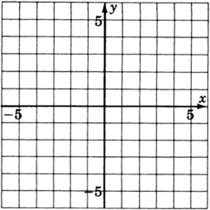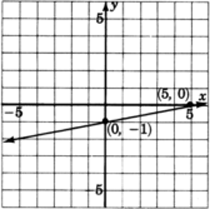##### Practice Problem $$\PageIndex{3}$$

$$x+2y=6$$##### Practice Problem $$\PageIndex{4}$$

$$2x+y=1$$A graph of a line passing through two points with coordinates zero, one and one, negative one.

## Slanted, Horizontal, and Vertical Lines

In all the graphs we have observed so far, the lines have been slanted. This will always be the case when both variables appear in the equation. If only one variable appears in the equation, then the line will be either vertical or horizontal. To see why, let’s consider a specific case:

Using the general form of a line, $$ax+by=c$$, we can produce an equation with exactly one variable by choosing $$a=0, b=5$$, and $$c=15$$. The equation $$ax+by=c$$ then becomes

$$0x+5y=15$$

Since $$0 \cdot (\text{Any Number}) = 0$$, the term $$0x$$ is $$0$$ for any number that is chosen for $$x$$.

Thus,

$$0x + 5y = 15$$

becomes $$0 + 5y = 15$$.

But, $$0$$ is the additive identity and $$0 + 5y = 5y$$.

$$5y = 15$$.

Then, solving for $$y$$ we get:

$$y = 3$$

This is an equation in which exactly one variable appears.

This means that regardless of which number we choose for $$x$$, the corresponding $$y$$-value is $$3$$. Since the $$y$$-value is always the same as we move from left-to-right through the $$x$$-values, the height of the line above the $$x$$-axis is always the same (in this case, 3 units). This type of line must be horizontal.

An argument similar to the one above will show that if the only variable that appears is $$x$$, we can expect to get a vertical line.

## Sample Set C

##### Example $$\PageIndex{6}$$

Graph $$y=4$$.
The only variable appearing is $$y$$. Regardless of which $$x$$-value we choose, the $$y$$-value is always $$4$$. All points with a $$y$$-value of $$4$$ satisfy the equation. Thus we get a horizontal line $$4$$ unit above the $$x$$-axis.

 $$x$$ $$y$$ $$(x, y)$$ $$−3$$ $$4$$ $$(−3, 4)$$ $$−2$$ $$4$$ $$(−2, 4)$$ $$−1$$ $$4$$ $$(−1, 4)$$ $$0$$ $$4$$ $$(0, 4)$$ $$1$$ $$4$$ $$(1, 4)$$ $$2$$ $$4$$ $$(2, 4)$$ $$3$$ $$4$$ $$(3, 4)$$ $$4$$ $$4$$ $$(4, 4)$$##### Example $$\PageIndex{7}$$

Graph $$x=−2$$.
The only variable that appears is $$x$$. Regardless of which $$y$$-value we choose, the $$x$$-value will always be $$−2$$. Thus, we get a vertical line two units to the left of the $$y$$-axis.

 $$x$$ $$y$$ $$(x,y)$$ $$−2$$ $$−4$$ $$(−2, −4)$$ $$−2$$ $$−3$$ $$(−2, −3)$$ $$−2$$ $$−2$$ $$(−2, −2)$$ $$−2$$ $$−1$$ $$(−2, −1)$$ $$−2$$ $$0$$ $$(−2, 0)$$ $$−2$$ $$1$$ $$(−2, 1)$$ $$−2$$ $$2$$ $$(−2, 0)$$ $$−2$$ $$3$$ $$(−2, 3)$$ $$−2$$ $$4$$ $$(−2, 4)$$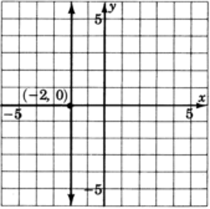## Practice Set C

##### Practice Problem $$\PageIndex{5}$$

Graph $$y=2$$.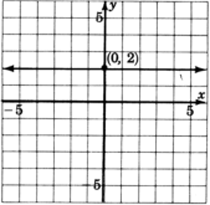##### Practice Problem $$\PageIndex{6}$$

Graph $$x=−4$$.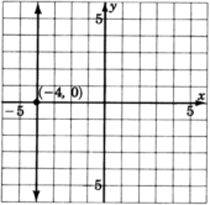Summarizing our results we can make the following observations:

1. When a linear equation in two variables is written in the form $$ax+by=c$$, we say it is written in general form.
2. To graph an equation in general form it is sometimes convenient to use the intercept method.
3. A linear equation in which both variables appear will graph as a slanted line.
4. A linear equation in which only one variable appears will graph as either a vertical or horizontal line.

$$x=a$$ graphs as a vertical line passing through $$a$$ on the $$x$$-axis.
$$y=b$$ graphs as a horizontal line passing through $$b$$ on the $$y$$-axis.

## Exercises

For the following problems, graph the equations.

##### Exercise $$\PageIndex{1}$$

$$−3x+y=−1$$##### Exercise $$\PageIndex{2}$$

$$3x−2y=6$$##### Exercise $$\PageIndex{3}$$

$$−2x+y=4$$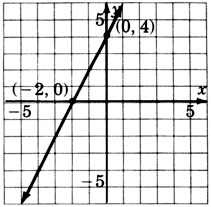##### Exercise $$\PageIndex{4}$$

$$x−3y=5$$##### Exercise $$\PageIndex{5}$$

$$2x−3y=6$$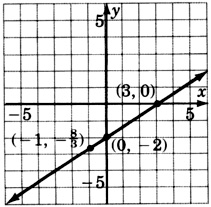##### Exercise $$\PageIndex{6}$$

$$2x+5y=10$$##### Exercise $$\PageIndex{7}$$

$$3(x−y)=9$$##### Exercise $$\PageIndex{8}$$

$$−2x+3y=−12$$##### Exercise $$\PageIndex{9}$$

$$y+x=1$$##### Exercise $$\PageIndex{10}$$

$$4y−x−12=0$$##### Exercise $$\PageIndex{11}$$

$$2x−y+4=0$$##### Exercise $$\PageIndex{12}$$

$$−2x+5y=0$$##### Exercise $$\PageIndex{13}$$

$$y−5x+4=0$$##### Exercise $$\PageIndex{14}$$

$$0x+y=3$$##### Exercise $$\PageIndex{15}$$

$$0x+2y=2$$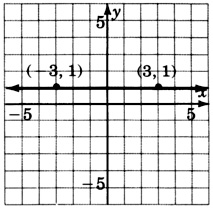##### Exercise $$\PageIndex{16}$$

$$0x + \dfrac{1}{4}y = 1$$##### Exercise $$\PageIndex{17}$$

$$4x+0y=16$$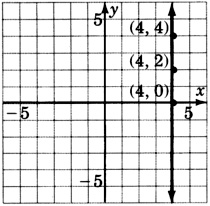##### Exercise $$\PageIndex{18}$$

$$\dfrac{1}{2}x + 0y = -1$$##### Exercise $$\PageIndex{19}$$

$$\dfrac{2}{3}x + 0y = 1$$$$x = \dfrac{3}{2}$$##### Exercise $$\PageIndex{20}$$

$$y=3$$##### Exercise $$\PageIndex{21}$$

$$y=−2$$$$y=−2$$##### Exercise $$\PageIndex{22}$$

$$−4y=20$$##### Exercise $$\PageIndex{23}$$

$$x=−4$$##### Exercise $$\PageIndex{24}$$

$$−3x=−9$$##### Exercise $$\PageIndex{25}$$

$$−x+4=0$$##### Exercise $$\PageIndex{26}$$

Construct the graph of all the points that have coordinates $$(a, a)$$, that is, for each point, the $$x$$ and $$y$$-values are the same.##### Exercise $$\PageIndex{27}$$

$$2.53x+4.77y=8.45$$##### Exercise $$\PageIndex{28}$$

$$1.96x+2.05y=6.55$$##### Exercise $$\PageIndex{29}$$

$$4.1x−6.6y=15.5$$##### Exercise $$\PageIndex{30}$$

$$626.01x−506.73y=2443.50$$## Exercises for Review

##### Exercise $$\PageIndex{31}$$

Name the property of real numbers that makes $$4+x=x+4$$ a true statement.

##### Exercise $$\PageIndex{32}$$

Supply the missing word. The absolute value of a number $$a$$, denoted $$|a|$$, is the from $$a$$ to $$0$$ on the number line.

##### Exercise $$\PageIndex{33}$$

Find the product $$(3x+2)(x−7)$$.

$$3x^2−19x−14$$

##### Exercise $$\PageIndex{34}$$

Solve the equation $$3[3(x−2)+4x]−24=0$$

##### Exercise $$\PageIndex{35}$$

Supply the missing word. The coordinate axes divide the plane into four equal regions called .